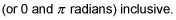##### Differential Equations For Dummies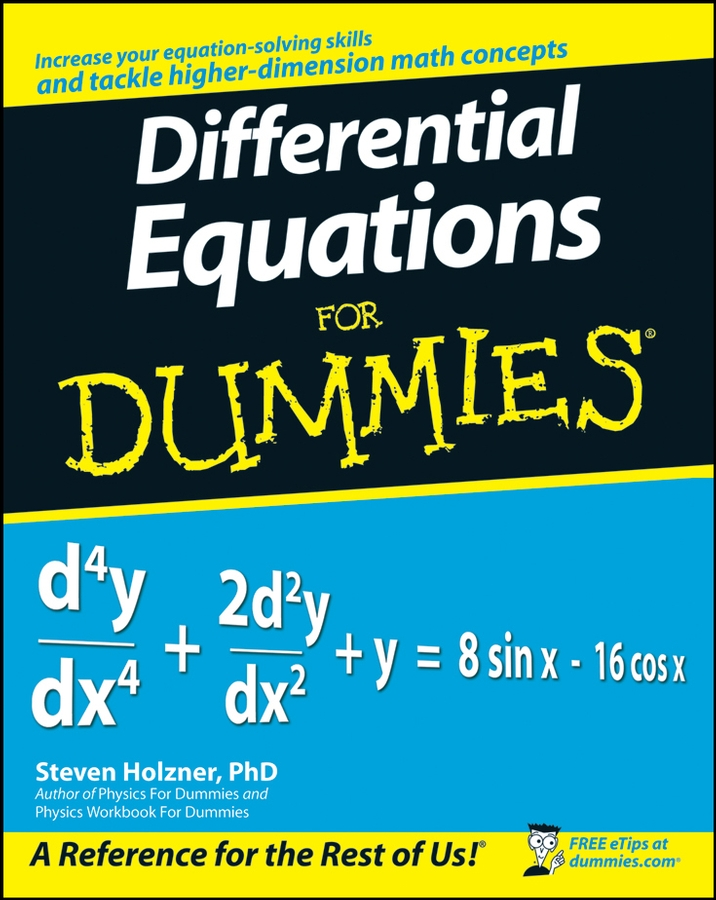Trigonometry is a very practical, real-world branch of mathematics, because it involves the measurement of lengths and angles. Surveyors use it when surveying property, making topographical maps, and so on, and the ancient Greeks, among others, used it for building, navigation, and astronomy.

Trigonometry comes up a lot in the study of calculus, so you may find the following practice problems to be helpful. (If you want to delve further into trig and functions, check out Calculus For Dummies, 2nd Edition, published by Wiley.)

## Practice questions

1. Use this right triangle,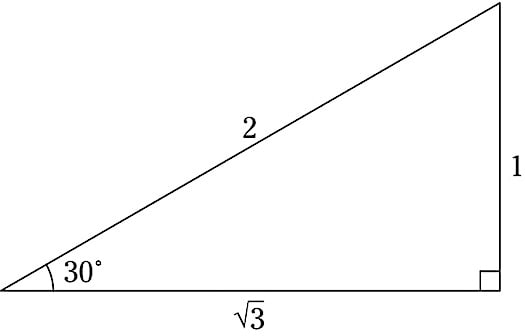to complete this table.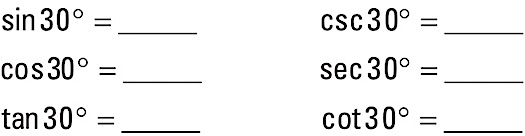2. Using this unit circle,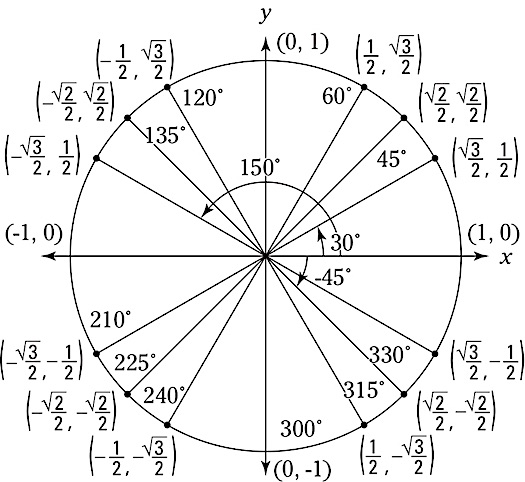complete the following table of inverse trig functions.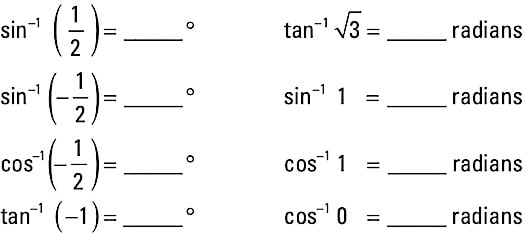1. The completed table: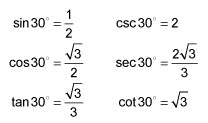2. The completed table of inverse trigonometric functions: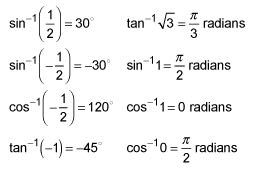Don’t forget—inverse sine and inverse tangent answers have to be between –90 degrees and 90 degrees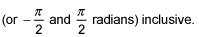inclusive. And inverse cosine answers must be between 0 degrees and 180 degrees